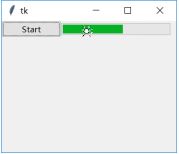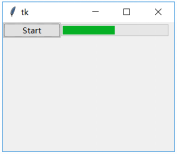# Python tkinter -- 第19章 进度条控件（Progressbar）

1）提示完成度

2）提示正在进行处理

19.1 属性

cursor 鼠标位于进度条内时的形状
length 进度条长度
maximum 进度条最大刻度值
mode 进度条的模式。有两种：‘determinate’和’indeterminate’
orient 进度条的方向，有HORIZONTAL 和VERTICAL两种
style 定义进度条的外观
takefocus 是否可以通过Tab获得输入焦点
variable 与进度条关联的变量。可以设置或获得进度条的当前值
value 设置或者获取进度条的当前值

19.1.1 cursor

import tkinter as tk
from tkinter import ttk
import time
def increment(*args):
for i in range(100):
p1["value"] = i+1
root.update()
time.sleep(0.1)
root = tk.Tk()
root.geometry('320x240')
p1 = ttk.Progressbar(root, length=200,cursor='spider',
mode="determinate",
orient=tk.HORIZONTAL)
p1.grid(row=1,column=1)
btn = ttk.Button(root,text="Start",command=increment)
btn.grid(row=1,column=0)
root.mainloop()19.1.2 length

import tkinter as tk
from tkinter import ttk
import time
def increment(*args):
for i in range(100):
p1["value"] = i+1
root.update()
time.sleep(0.1)
root = tk.Tk()
root.geometry('360x240')
p1 = ttk.Progressbar(root, length='2i', mode="determinate",
orient=tk.HORIZONTAL)
p1.grid(row=1,column=1)
btn = ttk.Button(root,text="Start",command=increment)
btn.grid(row=1,column=0)
root.mainloop()


19.1.3 maximum

import tkinter as tk
from tkinter import ttk
import time
def increment(*args):
for i in range(100):
p1["value"] = i+1
root.update()
time.sleep(0.1)
root = tk.Tk()
root.geometry('320x240')
p1 = ttk.Progressbar(root, length=200, mode="determinate",
maximum=200,orient=tk.HORIZONTAL)
p1.grid(row=1,column=1)
btn = ttk.Button(root,text="Start",command=increment)
btn.grid(row=1,column=0)
root.mainloop()19.1.4 mode

（1）determinate

（2）indeterminate

import tkinter as tk
from tkinter import ttk
import time
def increment(*args):
p1.start()
root = tk.Tk()
root.geometry('320x240')
p1 = ttk.Progressbar(root, length=200, mode="indeterminate",
maximum=200,orient=tk.HORIZONTAL)
p1.grid(row=1,column=1)
btn = ttk.Button(root,text="Start",command=increment)
btn.grid(row=1,column=0)
root.mainloop()


19.1.5 orient

19.1.6 style

19.1.7 takefocus

19.1.8 variable

19.1.9 value

19.2 函数

start(interval=None) 自动调整进度条的位置。通过启动一个循环定时事件，按照定义的步长调整进度条位置。定时器的间隔由interval参数来设定。间隔单位是毫秒.默认间隔是50毫秒。
step(amount=None) 每次调整进度条的步长，默认是1.0
stop() 停止定时器，停止进度条的自动调整

19.2.1 start(interval=None)

19.2.2 step(amount=None)

19.2.3 stop

import tkinter as tk
from tkinter import ttk
import time
def start(*args):
p1.start(30)
def stop(*args):
value=p1['value']
p1.stop()
p1['value']=value
root = tk.Tk()
root.geometry('320x240')
p1 = ttk.Progressbar(root, length=200,
mode="indeterminate",
orient=tk.HORIZONTAL)
p1.grid(row=1,column=0,columnspan=2)
btn_start = ttk.Button(root,text="开始",
command=start,width=10)
btn_start.grid(row=0,column=0)
btn_stop = ttk.Button(root,text="停止",
command=stop,width=10)
btn_stop.grid(row=0,column=1)
root.mainloop()©️2019 CSDN 皮肤主题: 大白 设计师: CSDN官方博客